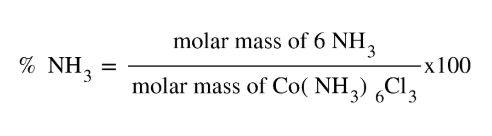# Problem: Determine the percent ammonia, NH3, in Co(NH3)6Cl3, to three significant figures.

###### FREE Expert Solution
• Determine the molar mass of the whole compound and then find the molar mass of 6 NHmolecules.
• Divide the MM of 6 NH3s over the total MM then multiply it by 100 to get the percent NH397% (89 ratings)###### Problem Details

Determine the percent ammonia, NH3, in Co(NH3)6Cl3, to three significant figures.